Monday 08th August 2022CBSE Guess > Papers > Important Questions > Class XI > 2010 > Maths > Maths By Mr. Anil Kumar Tondak CBSE CLASS XI
Sets Q. 1. Write the proper subsets of set A =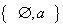Q. 2. Describe the set B =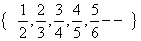Q. 3. Set A=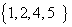, B=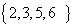and C=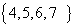find A-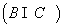Q. 4. Find the union of the following pair of sets A=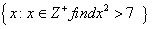; B=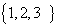Q. 5. Find the union and intersection of each of the following pairs of sets. (i) A={x: x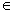Z+} ; B={x: xZ+ and x < 0} (ii) A={x : xN and 1